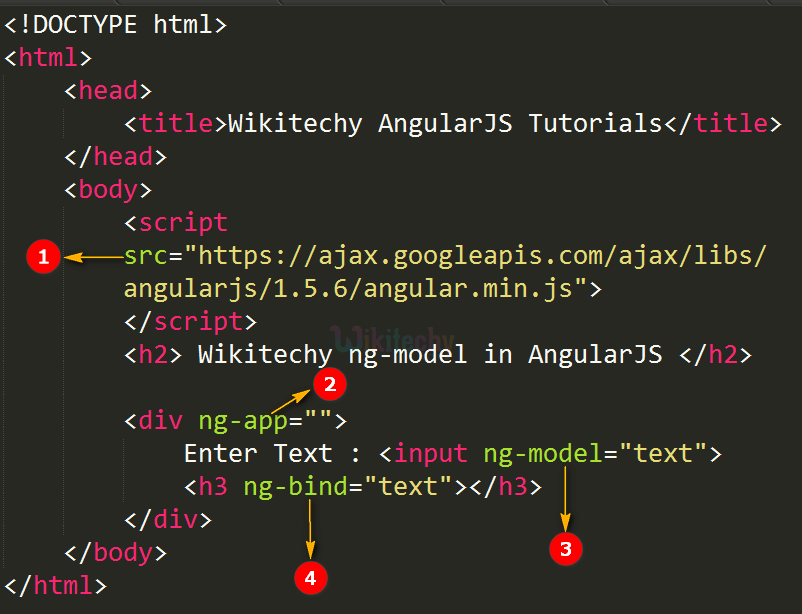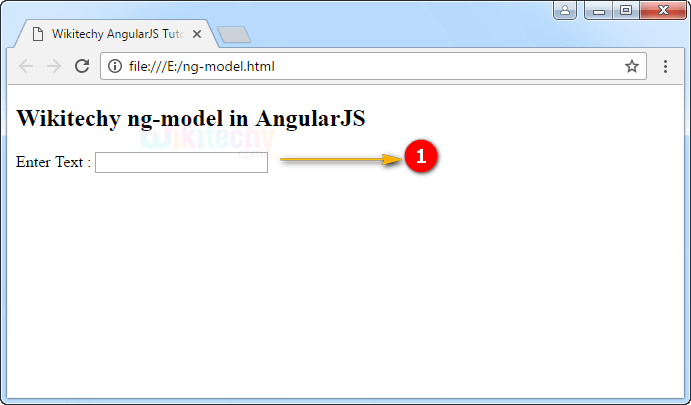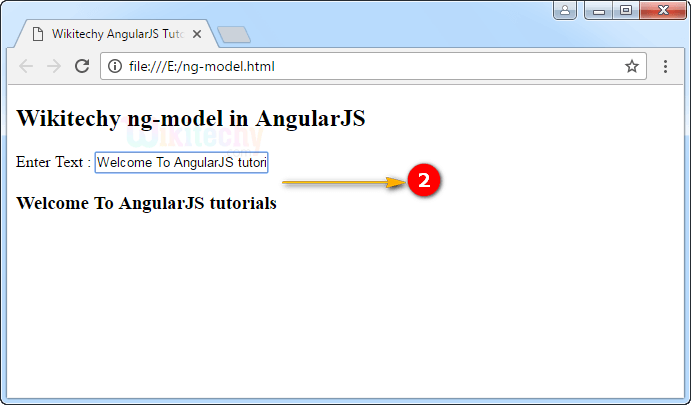# AngularJS ng-model

• ng-model directive is used to bind the following HTML controllers like inputs, textarea, select
• The ng-model directive stores the value of the input field from a variable.
• The ng-model supports two-way binding that means, if the user changed the input field value then display values will be auto-synchronized.
• The ng-model also supports
• To validate application data based on their types (email, number, required).
• To validate application data based on their status (invalid, touched, dirty, error).
• supports CSS classes for HTML elements.
• Bind HTML elements to HTML forms.

## Applies to:

Elements Attribute
<input> ng-model
<select> ng-model
<textarea> ng-model

## Parameter value for ng-model directive in AngularJS:

Value Description
name The input name stores/updates the value of the input field into/from a variable.

## ng-model directive declaration in AngularJS:

The ng-model bind an input field value to AngularJS application variable by using ng-model name as “text”.

## Code Explanation for ng-model directive in AngularJS:1. AngularJS is distributed as a JavaScript file, and can be added to a HTML page with a <script> tag.
2. The AngularJS application is defined by ng-app=" ". The application runs inside the <div> tag. It’s also used to define a <div> tag as a root element.
3. The ng-model bind an input field value to AngularJS application variable by using ng-model name as “text”.
4. The ng-bind is used to bind the content of the HTML <input> field value to application data.

## Sample Output for ng-model directive in AngularJS :1. The output displays the textbox.

2.3. The output shows a two-way binding. If the user type “Welcome To AngularJS tutorials” in textbox then the AngularJS using ng-model for displayed that text.